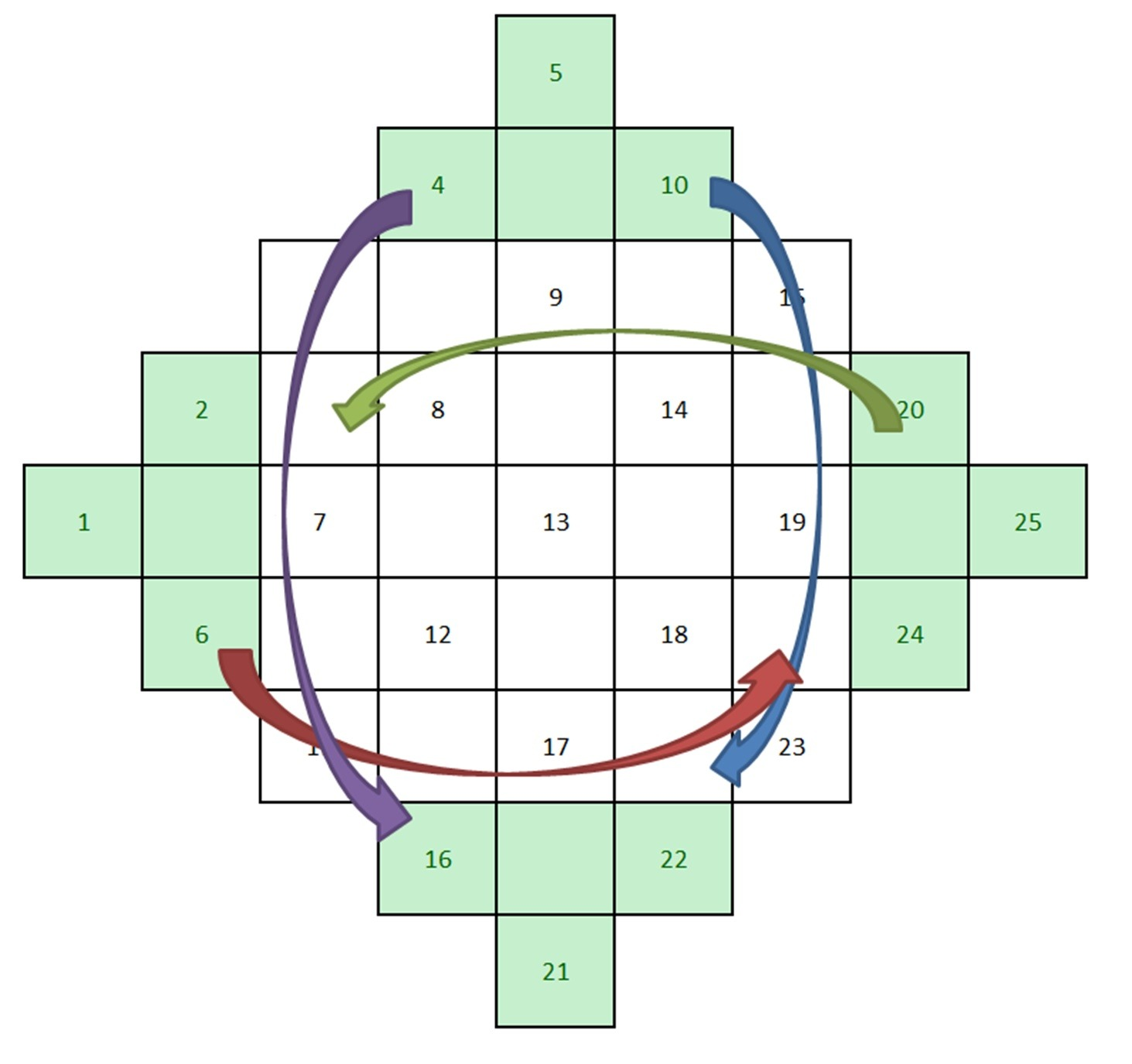# The Magic Square

Learn about the magic square. During the 14th century, Europe was devastated by the plague. Since medicine in the Middle Ages was extremely basic, they had no power over this terrible disease. People were terrified because they believed that Black Death was punishment from God.Image credit: Wikimedia Commons user Nicolas Poussin

They resorted to less verified sources and massively became superstitious. Here came the idea that mathematics can help prevent diseases and keep the devil at bay.

Some mathematicians created so-called magic square – a square table of order n containing numbers from 1 to n2 so that the sum of numbers in each row, column and both diagonals is the same. Soon enough, magic squares were everywhere.Image credit to Eve Andersson

## How do you calculate a magic square?

We will show you how to calculate a magic square.

## How to construct the magic squares of any order?

Order of the magic square represents the number of its rows or columns.

Construction of magic squares is divided into two different procedures:

• One where its order is odd
• One where it’s even

First, we’ll show the construction of magic squares with odd order.

Start with an empty square and draw a grid in it. For example, we’ll make a magic square whose order is 5.Divide every side on 5 parts and draw parallels to make a grid with 25 squares.

Now on every side of this square we’ll construct a “triangle” of 4 squares. You will see these in green.Now, starting from the top left diagonal, draw numbers from 1 to 25 in every other diagonal (including the added “triangles”).If the order is $n$, your last diagonal should end with n2. Now we should simply fill in the gaps. You take the numbers from those triangles and translate them vertically or horizontally to the furthest free place. If the number is placed on the top triangle, you’ll move it down. If it’s on the left, you’ll move it right and so on.And the final result is:Now we’ll show construction of magic squares of even order.

It is impossible to construct a magic square whose order is equal to 2. We’ll show how to construct a magic square whose order is equal to 4.

Draw a grid 4 x 4 and color them like this:Starting from the bottom right corner, write numbers from 1 to $n_2$.The numbers in the white cells are here to stay. But the numbers in the pink cells must be rearranged.

Now erase the number in the pink cells and start the counting again from the top right number corner.Now, the numbers in the white cells will remain as they were, and pink cells will take the numbers from the counting. This is the final result:Of course, you can test it out. The sum of all rows should be equal to the sum of all columns and the sum of diagonal numbers.